Share

# Draw a Line, Say Ab, Take a Point C Outside It. Through C, Draw a Line Parallel to Ab Using Ruler and Compasses Only - CBSE Class 7 - Mathematics

ConceptConstruction of a Line Parallel to a Given Line, Through a Point Not on the Line

#### Question

Draw a line, say AB, take a point C outside it. Through C, draw a line parallel to AB using ruler and compasses only

#### Solution

The steps of construction are as follows.

1) Draw a line AB. Take a point P on it. Take a point C outside this line. Join C to P.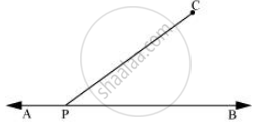2) Taking P as centre and with a convenient radius, draw an arc intersecting line AB at point D and PC at point E.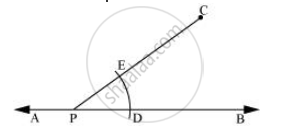3) Taking C as centre and with the same radius as before, draw an arc FG intersecting PC at H.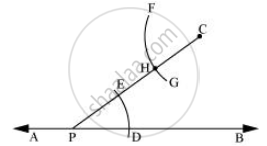4) Adjust the compasses up to the length of DE. Without changing the opening of compasses and taking H as the centre, draw an arc to intersect the previously drawn arc FG at point I.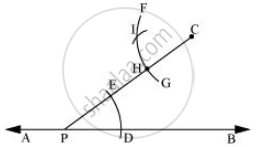5) Join the points C and I to draw a line 'l'.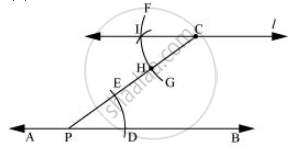This is the required line which is parallel to line AB.

Is there an error in this question or solution?

#### APPEARS IN

NCERT Solution for Mathematics for Class 7 (2018 to Current)
Chapter 10: Practical Geometry
Ex. 10.10 | Q: 1 | Page no. 196
Solution Draw a Line, Say Ab, Take a Point C Outside It. Through C, Draw a Line Parallel to Ab Using Ruler and Compasses Only Concept: Construction of a Line Parallel to a Given Line, Through a Point Not on the Line.
S